﻿ 基于数值模拟的气膜孔冷却效率经验公式的修正

# 基于数值模拟的气膜孔冷却效率经验公式的修正An Improved Empirical Formula for Film Hole Cooling Efficiency Based on Numerical Simulation

Abstract: Film cooling is a prospective cooling technique for maintaining and improving turbine working life with obvious advantages. The existing empirical formulas usually ignores the effect of the ratio of film hole length to the diameter L/D on the cooling effectiveness, but L/D is related to the surface inclination angle of film hole and the size of surface inclination angle of film hole greatly affects the flow pattern of the jet, and thus has a relatively large impact on the distribution of the cooling efficiency. The aim of this article is to construct an empirical formula through numerical experiments which can accurately predict the laterally averaged film cooling efficiency in the early stage of designing blade cooling structure. Therefore, the effects of film hole spacing, surface in-clination angle and blow ratio on the laterally averaged film cooling effectiveness were first inves-tigated by numerical simulations. The nonlinear fitting of the data was performed by using Matlab and were subsequently summarized as an empirical formula which is related to the above param-eters. Compared with the existing empirical formulas, the improved empirical formula can give a better prediction for cooling efficiency, whose coefficient of determination is greater than 0.9.

1. 引言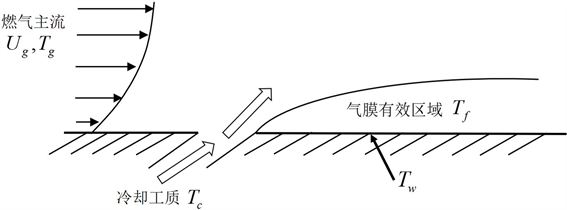Figure 1. Sketch of film cooling

2. 物理模型和计算方法

2.1. 计算域及网格划分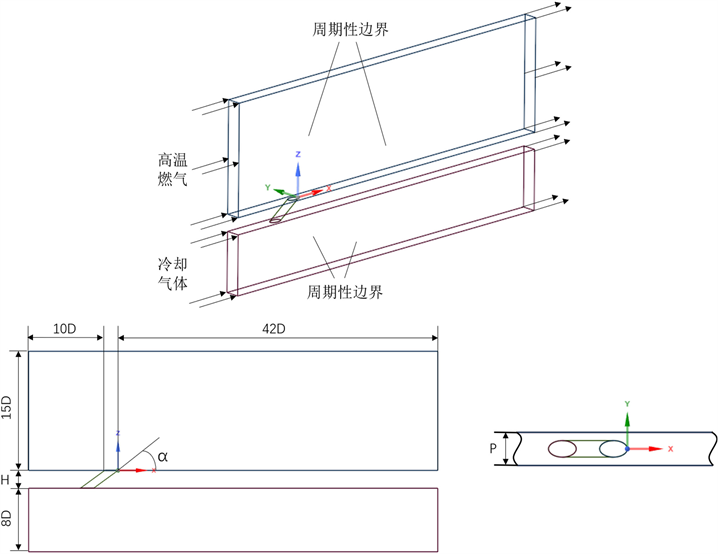Figure 2. Sketch of computational domain

2.2. 计算方法及边界条件设置Table 1. Boundary condition settings

2.3. 参数定义

$M=\left({\rho }_{c}{v}_{c}\right)/\left({\rho }_{\infty }{v}_{\infty }\right)$ (1)

$\eta =\left({T}_{\infty }-{T}_{aw}\right)/\left({T}_{\infty }-{T}_{c}\right)$ (2)

$\stackrel{¯}{\eta }=\frac{1}{n}\underset{i=1}{\overset{n}{\sum }}{\eta }_{i}$ (3)

${\stackrel{¯}{\eta }}_{area}=\frac{1}{m}\underset{i=1}{\overset{m}{\sum }}{\stackrel{¯}{\eta }}_{i}$ (4)

3. 计算结果与讨论

3.1. 计算结果验证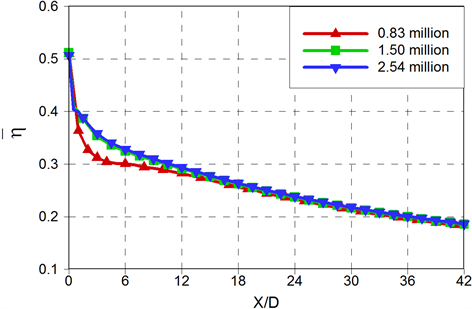Figure 3. Grid-independent verification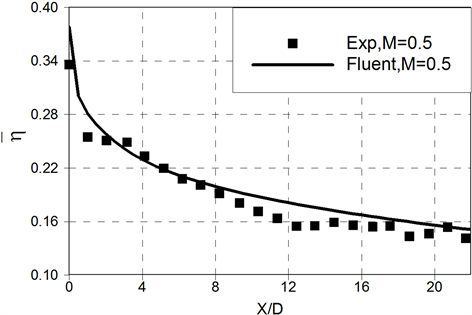Figure 4. Verification of calculation results

3.2. 几何参数与流动参数对气膜冷却的影响

$\stackrel{¯}{\eta }=f\left(P/D,\alpha ,M,X/D\right)$ (5)

3.2.1. 孔间距(P/D)的影响

$\stackrel{¯}{\eta }\propto \frac{1}{{\left(P/D\right)}^{n}}$ (6)Figure 5. The variation of laterally averaged film cooling efficiency $\stackrel{¯}{\eta }$ with the hole spacing

3.2.2. 气膜倾角(α)的影响Figure 6. Effect of α on laterally averaged film cooling efficiency $\stackrel{¯}{\eta }$ for cylindrical hole (P/D = 2, M = 0.6)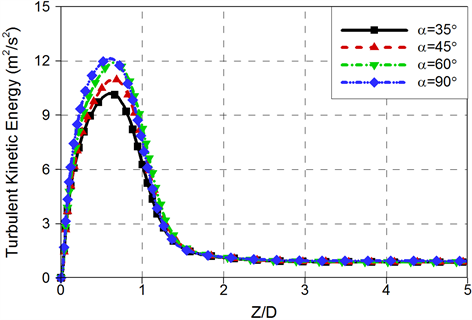Figure 7. The distribution of turbulent energy along the Z direction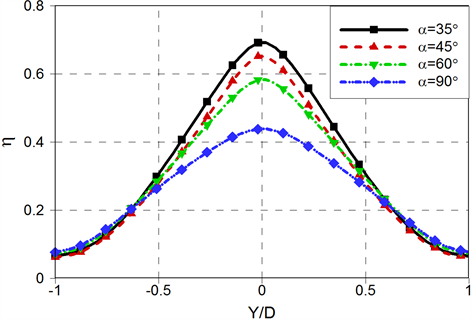Figure 8. The distribution of film cooling effectiveness along the Y direction

$\stackrel{¯}{\eta }\propto \frac{1}{{\left(\mathrm{sin}\alpha \right)}^{n}}$ (7)

3.2.3. 吹风比(M)的影响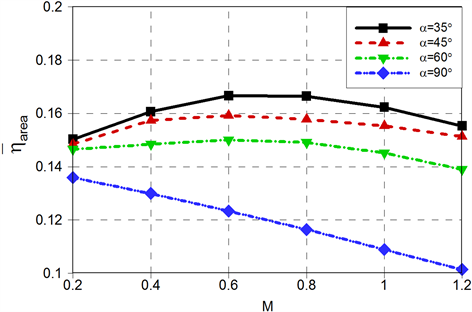Figure 9. Area-averaged film cooling effectiveness showing the blowing ratio effects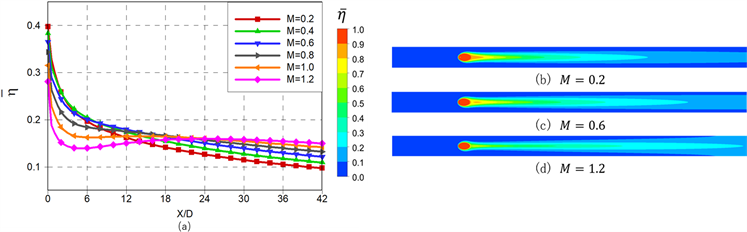Figure 10. Effect of blow ratio M on laterally averaged film cooling efficiency $\stackrel{¯}{\eta }$ (P/D = 2, α = 35˚)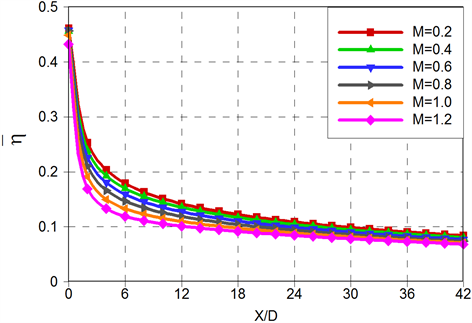Figure 11. Effect of blow ratio M on laterally averaged film cooling efficiency $\stackrel{¯}{\eta }$ (P/D = 3, α = 90˚)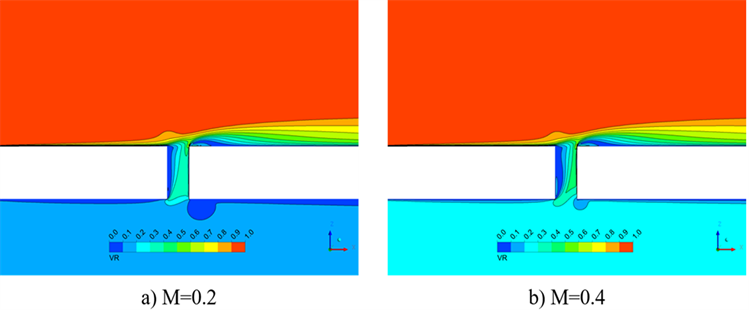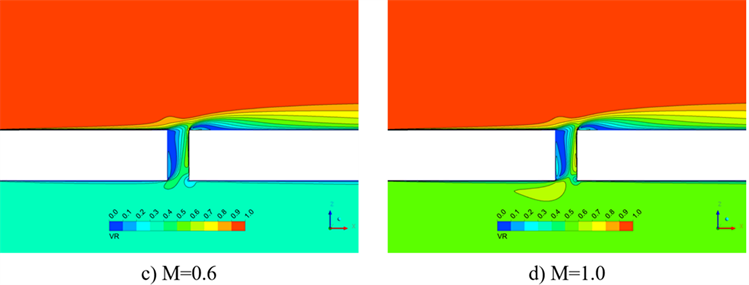Figure 12. The velocity contour of Y/D = 0 plane (P/D = 3, α = 90˚)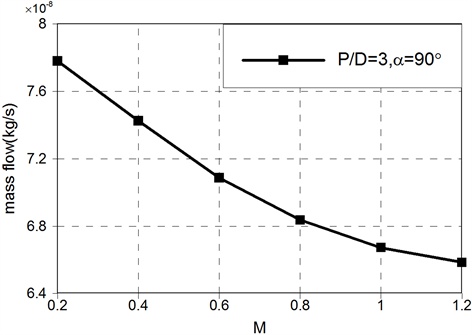Figure 13. The mass flow of the jet outlet changes with the blowing ratio (P/D = 3, α = 90˚)

3.3. 圆柱形孔气膜冷却效率关联式拟合

3.3.1. 吹风比较低时(0.2 ≤ M < 0.6)经验公式的拟合

$\stackrel{¯}{\eta }\propto {M}^{n}$ (8)

$\stackrel{¯}{\eta }\propto \frac{1}{{\left(X/D\right)}^{n}}$ (9)

$\stackrel{¯}{\eta }=\frac{1}{{C}_{5}{\left(P/D\right)}^{{C}_{1}}{\left(\mathrm{sin}\alpha \right)}^{{C}_{2}}{M}^{{C}_{3}}{\left(X/D\right)}^{{C}_{4}}+{C}_{6}{\left(P/D\right)}^{{C}_{7}}}$ (10)

$\stackrel{¯}{\eta }=\frac{{C}_{1}}{{\xi }^{{C}_{2}}}$ (11)

$\stackrel{¯}{\eta }=\frac{{C}_{1}}{{C}_{2}+\xi }$ (12)

$\stackrel{¯}{\eta }=\frac{{C}_{1}R{e}_{jet}^{0.2}}{{\xi }^{0.8}}$ (13)

$\stackrel{¯}{\eta }=\frac{{C}_{1}}{1+{\xi }^{0.8}}$ (14)

$\stackrel{¯}{\eta }=\frac{1}{P/t+{C}_{1}{M}^{{C}_{2}}{\xi }^{{C}_{3}}}$ (15)

$R{e}_{jet}=\frac{{\rho }_{jet}{U}_{jet}{S}_{e}}{{\mu }_{jet}}$ (16)Table 3. Empirical coefficients for Equations (10)-(15)

$回归平方和\left(SSR\right):\underset{i=1}{\overset{n}{\sum }}{\left({\stackrel{^}{y}}_{i}-\stackrel{¯}{y}\right)}^{2}$ (17)

$残差平方和\left(SSE\right):\underset{i=1}{\overset{n}{\sum }}{\left({y}_{i}-{\stackrel{^}{y}}_{i}\right)}^{2}$ (18)

$总离差平方和\left(SST\right):\underset{i=1}{\overset{n}{\sum }}{\left({y}_{i}-\stackrel{¯}{y}\right)}^{2}$ (19)

$决定系数:{R}^{2}=\frac{SSR}{SST}=1-\frac{SSE}{SST}$ (20)

R2的大小表征通过回归方程显示因变量的变化在自变量的变化中占据的百分比，即回归方程在多大程度上解释了因变量的变化，R2越接近1说明模型的拟合优度越高。图14展示了由式(10)至式(15)计算得到的M = 0.6时，α = 35˚，P/D = 3的圆柱形射流孔的展向平均气膜冷却效率与Fluent计算结果的对比，并且各经验公式的R2也标注在了图14中。可以看到本文拟定的式(10)的计算结果在 $\stackrel{¯}{\eta }$ 的数值以及分布趋势上与Fluent计算结果的吻合度要好于其它经验公式，除式(11)外的其余经验公式中，只有式(10)的R2大于0.9，但由于式(11)无法计算射流孔出口处(X/D = 0)的展向平均气膜冷却效率，因此式(10)更符合本文要求且拟合优度较高。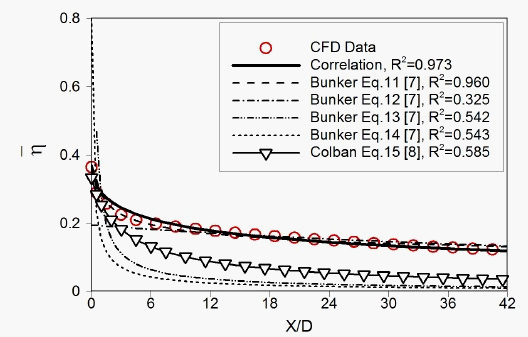Figure 14. Comparison of fitting effect of empirical formula under low blowing ratio (α = 35˚, P/D = 3˚, M = 0.6)

3.3.2. 吹风比较高时(0.6 < M ≤ 1.2)经验公式的拟合

$\stackrel{¯}{\eta }\propto \frac{1}{{M}^{n}}$ (21)

$\stackrel{¯}{\eta }=\frac{{C}_{1}{\left(X/D\right)}^{2}+{C}_{2}\left(X/D\right)+{C}_{3}}{{\left(X/D\right)}^{3}+{C}_{4}{\left(X/D\right)}^{2}+{C}_{5}\left(X/D\right)+{C}_{6}}+{C}_{7}{\left(P/D\right)}^{{C}_{8}}{M}^{{C}_{9}}{\left(\mathrm{sin}\alpha \right)}^{{C}_{10}}$ (22)Table 4. Empirical coefficients for the Equation (23)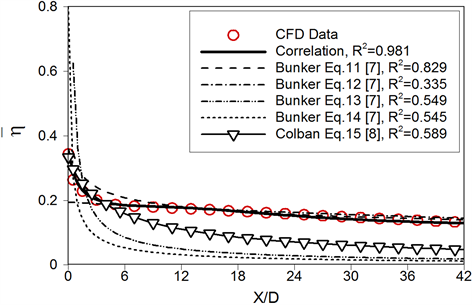Figure 15. Comparison of the fitting effect of the empirical formula when the blowing ratio is 0.8 (α = 35˚, P/D = 3)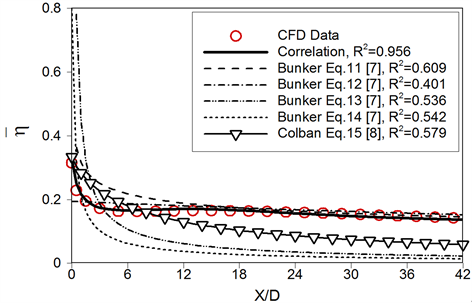Figure 16. Comparison of the fitting effect of the empirical formula when the blowing ratio is 1.0 (α = 35˚, P/D = 3)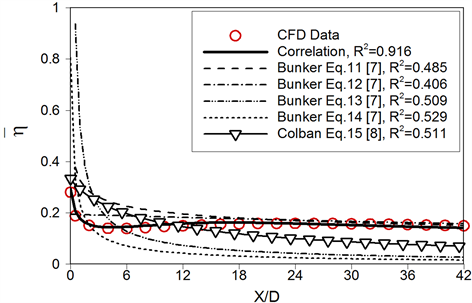Figure 17. Comparison of the fitting effect of the empirical formula when the blowing ratio is 1.2 (α = 35˚, P/D = 3)

4. 结论

1) 当气膜孔间距较小时，射流孔间的冷却气体发生强烈的相互作用，冷却气体分布均匀，整体冷却效率较高；当孔间距较大时，冷却气体之间的相互作用减弱，导致各射流孔间会随着孔间距的增大，出现不能被气膜覆盖的区域，气膜冷却效率随之下降。

2) 当气膜倾角较小时，冷却气体从射流孔射出后，能够很好地贴紧壁面，使射流孔出口附近的冷却效率增高。适当地增大吹风比，有益于增大冷却气体在射流孔出口处的流量，使得下游的覆盖区域变大，冷却效果变好；但过大的吹风比会使得冷却气体在射流孔出口附近脱离壁面，导致射流孔出口处冷却效率降低，而在远离射流孔出口处，脱离壁面的冷却气体会发生再附着，使气膜冷却效率回升。当气膜倾角较大时，冷却气体在射流孔出口处很容易脱离壁面，导致射流孔出口附近的冷却效率较低。随着气膜倾角的增大，冷却气体在射流孔内背离射流孔出口处出现的旋涡区域也逐渐增大，阻碍冷却气体流出，使射流孔出口处的流量增大趋势逐渐减弱，甚至出现流量逐渐减小的情况。在较高吹风比下，冷却气体被主流很快压回壁面，在尾缘处不会发生再附着的现象。

3) 提出了两组气膜冷却效率经验公式，分别适用于吹风比在0.2 ≤ M ≤ 0.6和0.6 < M ≤ 1.2的区间，引入了气膜孔倾角这一几何参数对冷却效率的影响。由两组经验公式预测的冷却效率与数值计算吻合较好，较前人的经验公式均有所改进，R2值达到了0.9以上，说明具有较高的拟合优度。

 戴萍, 林枫. 燃气轮机叶片气膜冷却研究进展[J]. 热能动力工程, 2009(1): 5-10, 143.

 乔日平, 朱励. 不同孔间距气膜冷却的数值模拟[J]. 东北电力大学学报, 2009, 29(1): 36-39.

 郭婷婷, 金建国, 李少华, 等. 不同出射角度对气膜冷却流场的影响[J]. 中国电机工程学报, 2006, 26(16): 117-121.

 李少华, 宋东辉, 刘建红, 等. 不同孔型平板气膜冷却的数值模拟[J]. 中国电机工程学报, 2006(17): 114-118.

 韩振兴, 刘石, 刘建军, 等. 吹风比对燃汽轮机平板气膜冷却特性影响的实验研究[J]. 中国电机工程学报, 2005, 25(18): 91-96.

 Baldauf, S., Schleurlen, M., Schulz, A. and Wittig, S. (2002) Correlation of Film Cooling Effectiveness From Thermographic Measurements at Engine Like Conditions. ASME Turbo Expo 2002: Power for Land, Sea, and Air, Amsterdam, 3-6 June 2002, ASME Paper No. GT-2002-30180, 149-162.
https://doi.org/10.1115/GT2002-30180

 Bunker, R.S. (2005) Turbine Cooling Design Analysis. Gas Turbine Handbook, Department of Energy, Washington, DC, Sec. 4.2.1.

 Colban, W., Thole, K.A. and Bogard, D. (2011) A Film Cooling Correlation for Shaped Holes on a Flat-Plate Surface. Journal of Turbomachinery, 133, Article ID: 011002.
https://doi.org/10.1115/1.4002064

 Chen, A.F., Li, S.J. and Han, J.C. (2015) Film Cooling for Cylindrical and Fan-Shaped Holes Using Pressure-Sensitive Paint Measurement Technique. Journal of Thermophysics and Heat Transfer, 29, 1-10.

 Zhang, H., Li, Y., Chen, Z., et al. (2019) Multi-Fidelity Model Based Optimization of Shaped Film Cooling Hole and Experimental Validation. International Journal of Heat and Mass Transfer, 132, 118-129.
https://doi.org/10.1016/j.ijheatmasstransfer.2018.11.156

 Wang, N., Zhang, M., Shiau, C.C., et al. (2019) Film Cooling Effectiveness from Two Rows of Compound Angled Cylindrical Holes Using Pressure-Sensitive Paint Technique. Journal of Heat Transfer, 141, Article ID: 042202.

 王文萍, 邹歆, 孙鹏, 等. 高温平板气膜冷却耦合换热实验与计算[J]. 清华大学学报(自然科学版), 2010(12): 90-95.

 Walters, D.K. (2000) Impact of Film-Cooling Jets on Turbine Aerodynamic Losses. Journal of Turbomachinery, 122, 537-545.
https://doi.org/10.1115/1.1303818

 刘存良, 朱惠人, 白江涛. 收缩–扩张形气膜孔提高气膜冷却效率的机理研究[J]. 航空动力学报, 2008, 23(4): 598-604.

 刘捷, 韩振兴, 刘建军, 等. 射流注入角对平板气膜冷却特性影响的实验研究[J]. 工程热物理学报, 2007, 28(3): 409-411.

Top